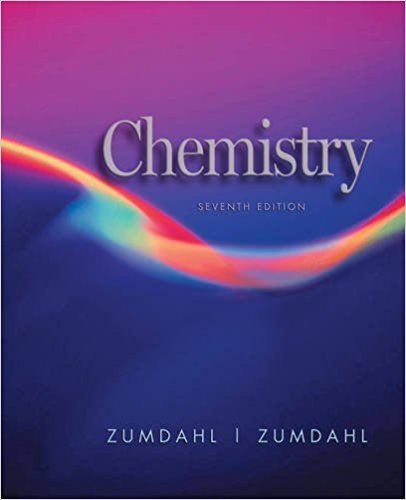×
×

Textbooks / Chemistry / Chemistry 7

# Chemistry 7th Edition - Solutions by Chapter## Full solutions for Chemistry | 7th Edition

ISBN: 9780618528448Chemistry | 7th Edition - Solutions by Chapter

Solutions by Chapter
4 5 0 433 Reviews
##### ISBN: 9780618528448

Chemistry was written by and is associated to the ISBN: 9780618528448. The full step-by-step solution to problem in Chemistry were answered by , our top Chemistry solution expert on 12/23/17, 04:50PM. This textbook survival guide was created for the textbook: Chemistry, edition: 7. This expansive textbook survival guide covers the following chapters: 22. Since problems from 22 chapters in Chemistry have been answered, more than 43222 students have viewed full step-by-step answer.

Key Chemistry Terms and definitions covered in this textbook

An addition reaction in which two groups are installed on opposite sides of a p bond.

• beta particles.

See beta rays.

• Cis, trans isomers

Stereoisomers that have the same connectivity but a different arrangement of their atoms in space as a result of the presence of either a ring or a carboncarbon double bond.

Addition of a nucleophile to the b-carbon of an a,b-unsaturated carbonyl compound. (Section 20.2A) Addition to carbons 1 and 4 of a conjugated diene.

• crystalline solid.

A solid that possesses rigid and long-range order; its atoms, molecules, or ions occupy specific positions. (11.4)

A cyclohexene resulting from the cycloaddition reaction of a diene and a dienophile.

• effusion

The escape of a gas through an orifice or hole. (Section 10.8)

• fossil fuels

Coal, oil, and natural gas, which are presently our major sources of energy. (Section 5.8)

• geometric isomerism

A form of isomerism in which compounds with the same type and number of atoms and the same chemical bonds have different spatial arrangements of these atoms and bonds. (Sections 23.4 and 24.4)

• ideal-gas equation

An equation of state for gases that embodies Boyle’s law, Charles’s law, and Avogadro’s hypothesis in the form PV = nRT. (Section 10.4)

• Infrared (IR) spectroscopy

A spectroscopic technique in which a compound is irradiated with infrared radiation, absorption of which causes covalent bonds to change from a lower vibration state to a higher one. Infrared spectroscopy is particularly valuable for determining the kinds of functional groups present in a molecule.

• law of constant composition

A law that states that the elemental composition of a pure compound is always the same, regardless of its source; also called the law of definite proportions. (Section 1.2)

• mass number

The sum of the number of protons and neutrons in the nucleus of a particular atom. (Section 2.3)

• Melt transition (Tm)

The temperature at which crystalline regions of a polymer melt.

• microstate

The state of a system at a particular instant; one of many possible energetically equivalent ways to arrange the components of a system to achieve a particular state. (Section 19.3)

• mineral

A solid, inorganic substance occurring in nature, such as calcium carbonate, which occurs as calcite. (Section 23.1)

• net ionic equation

A chemical equation for a solution reaction in which soluble strong electrolytes are written as ions and spectator ions are omitted. (Section 4.2)

• polyatomic ion

An electrically charged group of two or more atoms. (Section 2.7)

• steroids

Lipids that are based on a tetracyclic ring system involving three six-membered rings and one five-membered ring. Cholesterol is an example.

• thiols

Compounds containing a mercapto group (SH).

×